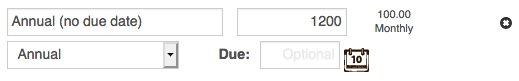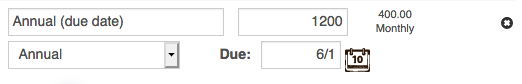# How are Monthly budgeted amounts for Annual / Goal Envelopes calculated?

The suggested Fill amounts for Annual and Goal Envelopes differ depending on whether or not they are assigned a Due Date.

Annual Envelopes without a Due Date calculate their Fill amounts based on the Annual budgeted amount divided by the number of budget periods in a year. For example, if you are using a Monthly primary budget period, your Monthly Fill amount will be your Annual budgeted amount divided by 12.Goal Envelopes without a Due Date will always have a Fill amount of 0.

Annual and Goal Envelopes that have Due Dates calculate their Fill amounts very similarly. Both will calculate based on the Annual/Goal budgeted amount minus the amount that’s already been Filled or Transferred into that Envelope, divided by the number of budget periods until the Due Date.

For an example, imagine you’re using a Monthly budget period, and have an Annual/Goal Envelope with a Annual/Goal budgeted amount of 1200, a Due Date of 4 months from the current date, and have never Filled or Transferred money into that Envelope. The Fill calculation for that Envelope would be (1200 – 0) / 4 = 400.Now imagine the same Envelope, except you’re using Semi-Monthly budget period, and you’ve previously Filled the Envelope by 50. The Fill calculation for that Envelope would be (1200 – 50) / 8 = 143.75.

It’s important to note that the Fill amount calculations do not take current balance into account, so even if you’ve spent some of the money you previously saved, that previously saved money is still used for the calculations.